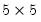Command Reference : Matrix Language Reference

 @vech
Vectorize (stack columns of) lower triangle of matrix.
Syntax: @vech(m)
m: matrix, sym
Return: vector
Creates a vector from the columns of the lower triangle of the source square matrix m stacked on top of each another.
The vector has the same number of elements as the source matrix has in its lower triangle.
Examples
sym s1 = @mrnd(5, 5)
vector v1 = @vech(m1)
creates asym matrix S1 and stacks the elements in its lower triangle in a 15 element vector V1.
If M is a matrix,
scalar is_symm = @vech(M)=@vech(M.@t)
tests for symmetry by seeing whether the lower and upper triangles of the matrix are equal. If the upper and lower elements triangles are the same, then the equality test will return 1, otherwise, it will return 0.
Cross-references